Computational Biology

Proteins are the molecular-scale machines which conduct and mediate the fundamental processes of life. The beautiful and diverse ways in which they perform their tasks are a perk of their incredible chemical and structural diversity.

While DNA and RNA only have four different building blocks, proteins employ at least 20 amino acid building blocks named for most of the letters in the alphabet ($A, C, D, E,$ etc.). Long linear chains of amino acids fold up into complex and compact structures.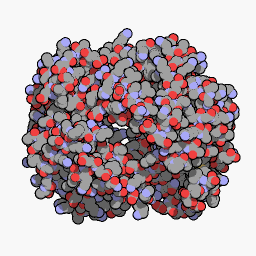Human Myoglobin. PDB: 3RGK Structure: QuteMol

Designing and manufacturing atomic-scale structures like proteins is well beyond our current technology, so understanding how proteins fold is a giant unsolved problem in computational biology.

Protein Origami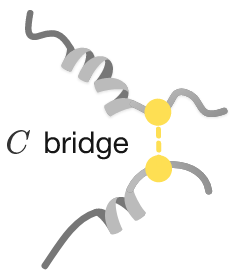Early in the study of protein structures, most scientists believed that the 3D folded structure of proteins was "stapled" together in several locations by strong chemical bonds called disulfide bridges. Much like base-pairs which hold together double-stranded DNA and RNA, it was thought that a certain amino acid called cysteine $($or $C$ for short$)$ could come together like velcro to form strong bonds, the only chemical bonds that were known to occur in folded proteins.

Future Nobel prize winner Christian Anfinsen used an active protein called an enzyme to study folding. This enzyme contained eight $C$ amino acids which could form four bridges. Though he could not observe the exact atomic structure of the protein as it folded, he knew the protein was in the right arrangement when it performed its biological function: chopping up RNA.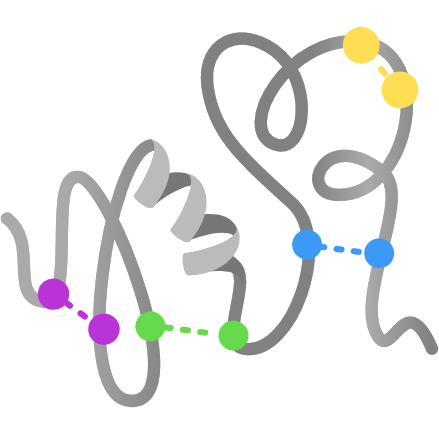Considering only these eight $C$ amino acids, how many possible arrangements of four bridges can appear in this enzyme?

Protein Origami

Even though the $C$ amino acids could form 105 different bridge arrangements, Anfinsen observed one arrangement well over $99 \%$ of the time. He called this the native fold. How the protein selected this correct arrangement was unknown.

Proteins and their $C$ bridges can be unfolded by heating and adding chemicals like alcohol which interfere with biological structures. When Anfinsen unfolded the enzyme, all of the bridges were broken and it became non-functional—it wouldn’t chop up any RNA.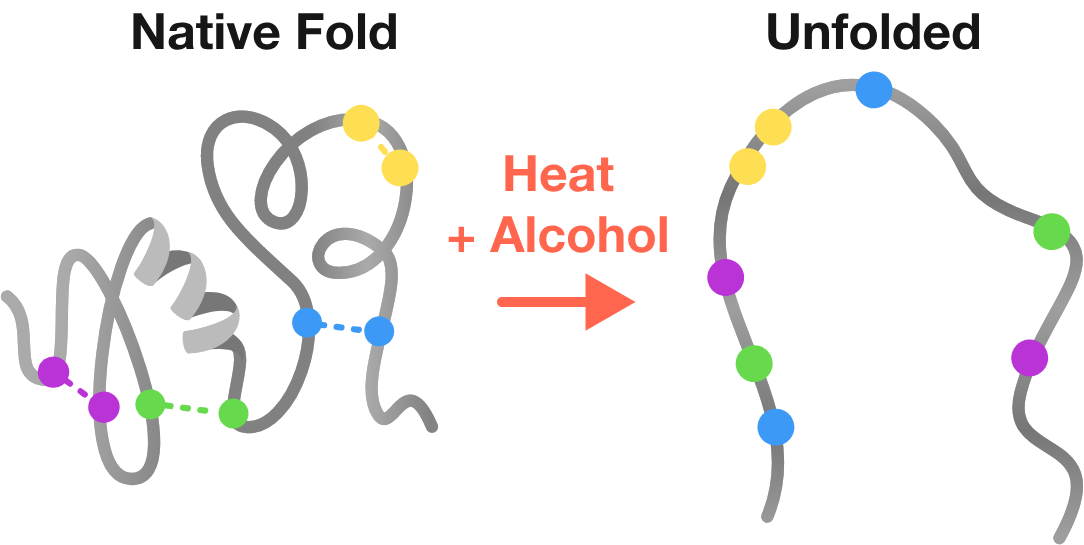What could Anfinsen conclude about the role of structure in protein function?

Protein Origami

When he cooled the protein in alcohol, all four $C$ bridges reformed, but instead of selecting the native fold, they occupied each of the 105 bridge combinations roughly equally. This mixture of differently arranged proteins demonstrated only about $1\%$ of the RNA chopping activity expected from the pure protein.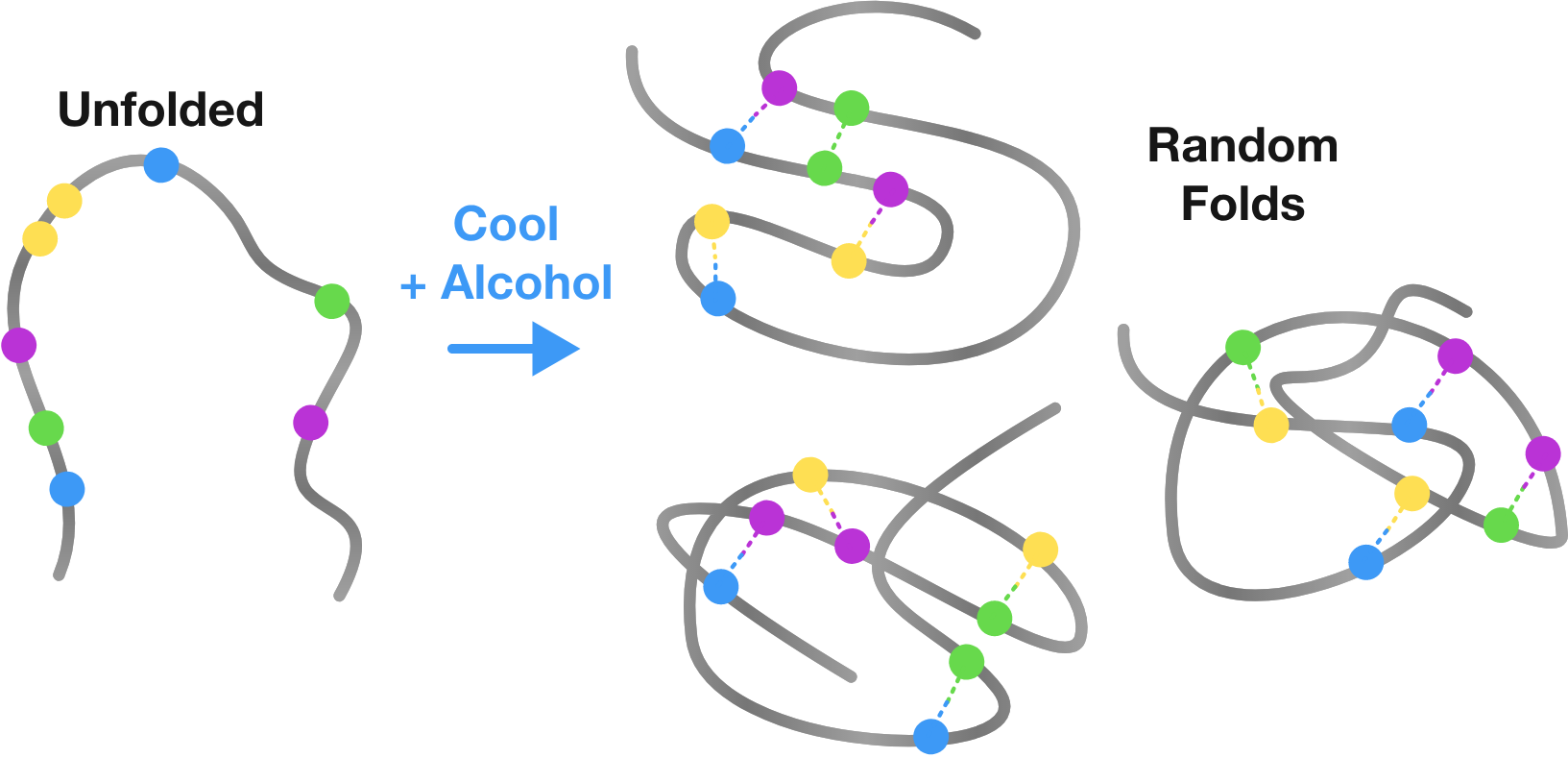The alcohol seemed to be interfering with the enzyme finding the correct configuration of bridges.

Protein Origami

The randomly configured enzymes had almost no useful activity since only a very small fraction would be in the native fold randomly. Anfinsen then removed the alcohol from this random population of proteins and returned the environment back to a more familiar salty water that's found inside cells.

He observed that the enzyme's RNA chopping activity slowly increased back to $100 \%$, as $C$ bridges broke apart and tended to reform into the native fold all on their own.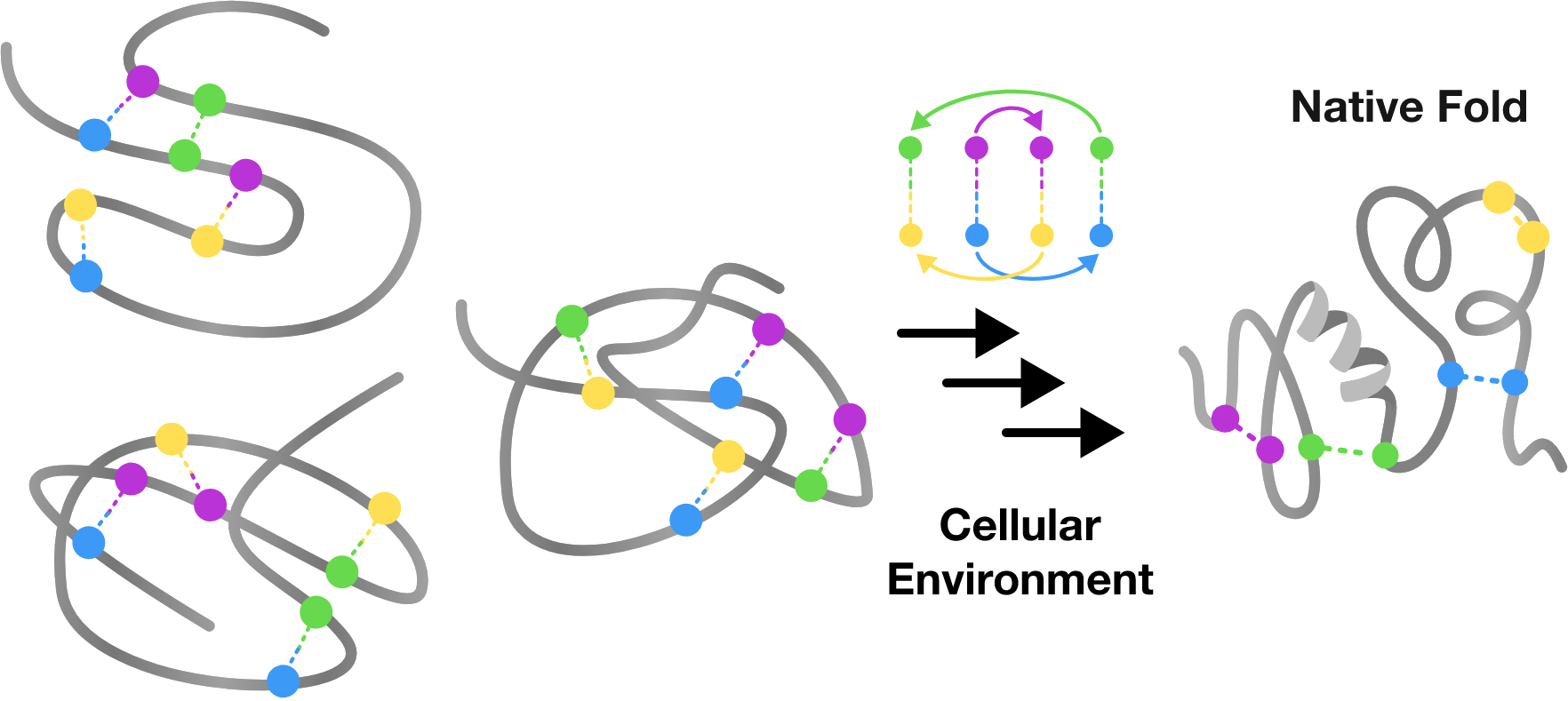What does this suggest about the role of $C$ bridges in determining protein structure?

Protein Origami

Anfinsen's experiment indicated that the arrangement of $C$ bridges does not fully determine protein structure. The complete story involves some other force that pushes the protein towards its native fold. If a protein is forced towards its native fold, that means it must have lower energy. Just as the force of gravity pulls a ball to lower gravitational potential energy, the forces in play in protein folding pull a protein towards the lowest energy fold.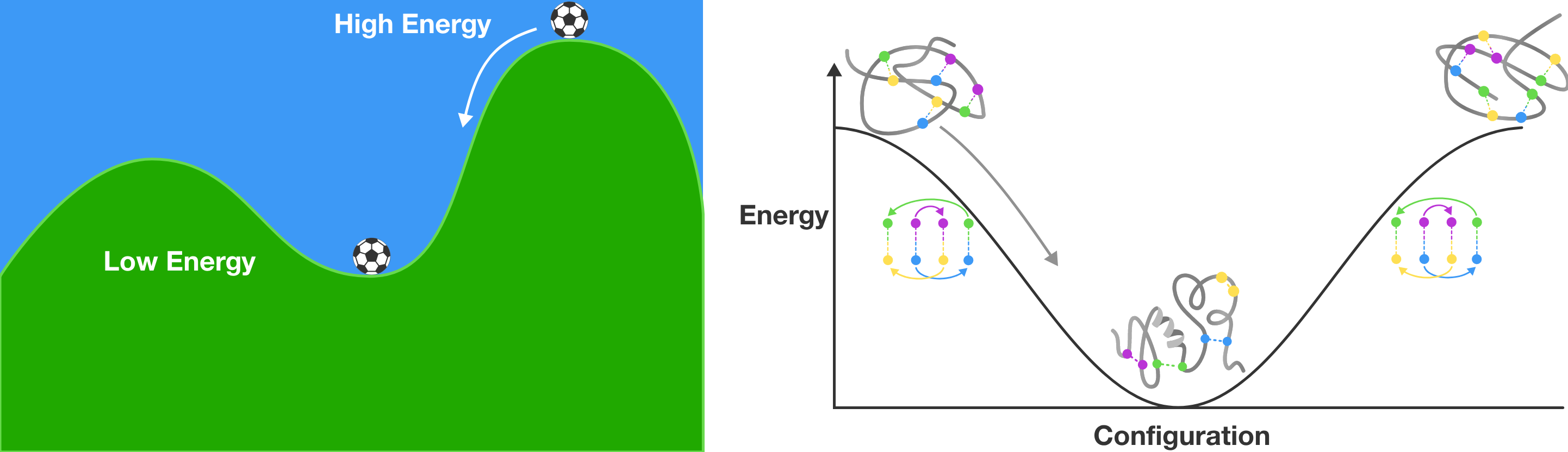The exact identity of the forces biasing the protein structure towards its native fold was unknown at the time, but Anfinsen proposed,

"Interactions between the functional groups of the side chains may exert, by a concerted action, a powerful set of forces that allow a significant fraction of molecules to favor a configuration resembling that of the native fold, even in the absence of stabilizing [bridges]."

This is the basis of the thermodynamic hypothesis, which states that the native structure of a protein is determined by interactions between all of the protein's amino acids which act in concert with the environment to form its native fold.

Protein Origami

Just like DNA and RNA, lower energy protein structures have more bonds. But $C$ bridges are only one of many types of bonds that form as proteins fold. Some bridge configurations are lower energy than others because they allow many other bonds to form. In the Folding chapter, we'll use information theory to tease out the other bonds involved, including hydrogen bonds, salt bridges, and $\pi$-$\pi$ stacking between two or more amino acids.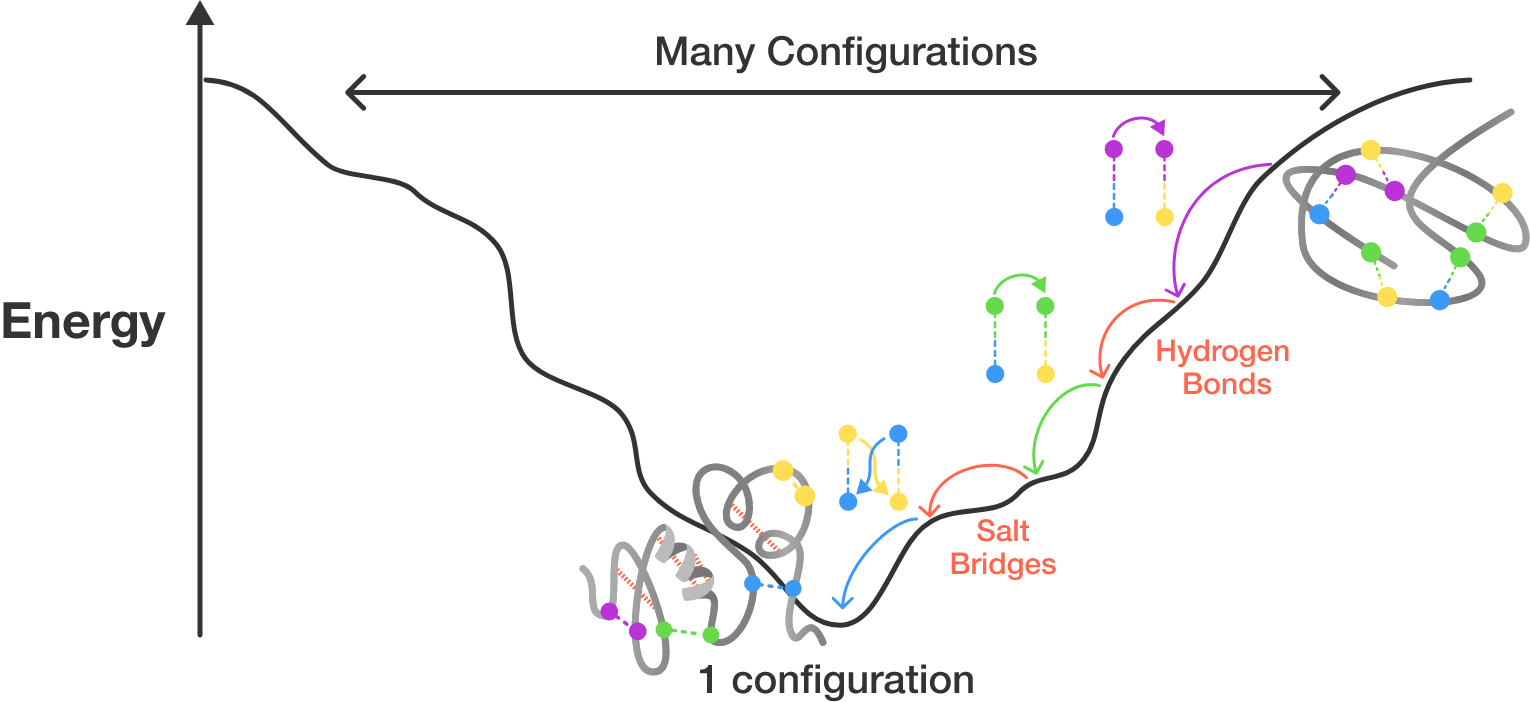Considering only four $C$ bridges, there are 105 possible bridge configurations, only one of which is the native fold. If we want to find the lowest energy configuration, we could count the total number of bonds in each of the 105 configurations. Then the lowest energy configuration is the one with the highest number of bonds $($including $C$ bridges and all other types$).$

Why might this straightforward approach be doomed to failure?

Protein Origami

The immensity of possible interactions and configurations of proteins is intimidating, but it turns out that proteins occupy only tiny islands in a vast sea of possible configurations. By finding simple patterns in protein configurations, we can focus only on these islands and make the protein folding problem much more tractable.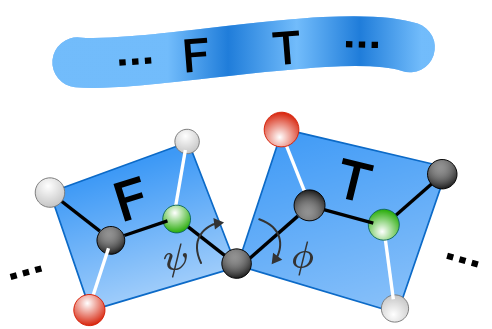A protein is a linear sequence of amino acids, each of which is constrained to a plane. The bonds connecting each amino acid are free to rotate, so the configuration of two adjacent amino acids can be described using their dihedral angles. Naively, every pair of amino acids could have angles anywhere between $-\pi$ and $\pi$ radians, but that’s not what we find.

The Python environment below takes in the 3D structure of hemoglobin and measures the dihedral angles between every amino acid. The program outputs a scatter plot of all the different orientations in the protein.

# Import Biopython, Matplotlib and NumPy libraries
import Bio.PDB
import matplotlib.pyplot as plt
import matplotlib.ticker as tck
import numpy as np

# Import the Hemoglobin coordinates file from the Protein Data Bank.
structure = Bio.PDB.PDBParser(QUIET=True).get_structure('Hemoglobin', 'data/1a3n.pdb')

# Define a function to build a model of the protein from the coordinates
def build_model(structure):
angles = []
for model in structure:
for chain in model:
polypeptides = Bio.PDB.CaPPBuilder().build_peptides(chain)
for poly_index, poly in enumerate(polypeptides):
phi_psi = poly.get_phi_psi_list()
for res_index, residue in enumerate(poly):
phi, psi = phi_psi[res_index]
if phi and psi:
angles.append(['Hemoglobin', str(chain.id), residue.resname,
residue.id, phi / np.pi, psi / np.pi])
return np.array(angles)

# Run our function.
angles = build_model(structure)
phi = np.array(angles[:, 4], dtype='float')
psi = np.array(angles[:, 5], dtype='float')

# Plot the results
f, ax = plt.subplots(1)

ax.xaxis.set_major_formatter(tck.FormatStrFormatter('%g $\pi$'))
ax.xaxis.set_major_locator(tck.MultipleLocator(base=0.5))
ax.yaxis.set_major_formatter(tck.FormatStrFormatter('%g $\pi$'))
ax.yaxis.set_major_locator(tck.MultipleLocator(base=1))
plt.ylim((-1, 1))
plt.xlim((-1, 1))
plt.xlabel('$\phi$')
plt.ylabel('$\psi$')
ax.scatter(phi, psi)

plt.savefig("Islands.png", format="png")

Import the Hemoglobin coordinates file from the Protein Data Bank.

Parse the protein databank file and extract the protein sequence, to determine geometry one amino acid at a time.

Extract $\phi$ and $\psi$ angles for each amino acid.

Generate an array with the attributes of each amino acid in the protein.

Generate a scatter plot of the $\phi$ and $\psi$ angles of every amino acid in the protein.

Python 3
You need to be connected to run code

Protein Origami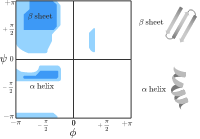The two largest islands in protein configurational space are called alpha helices and beta sheets for the characteristic shapes of their folds. Together with several other common motifs, these are known as protein secondary structures. Though an amino acid could theoretically occupy a configuration with an arbitrary torsion angle, finding patterns of different configurations can help us improve our "guesses" of possible protein structures.

Not only do different secondary structures appear as islands in plot of possible dihedral angles, the different amino acids tend to occupy one secondary structure over the others due to their unique chemical properties. Knowledge of these patterns can help us even more to find the native fold.

We will find these patterns by mining protein structures. The Python environment below has been prepared to generate torsion distributions for three different amino acid patterns Glycine $(G)$, Alanine $(A)$, and any amino acid preceding a Proline $(P).$ You'll learn how to use Python to perform analysis on patterns of protein folding in an upcoming chapter.

What conclusions can you make about the structural tendencies of these amino acids using the "map" of amino acid configurations above?

# Import Biopython, Matplotlib and NumPy libraries
import Bio.PDB
import matplotlib.pyplot as plt
import matplotlib.ticker as tck
import numpy as np

# Import the Hemoglobin coordinates file from the Protein Data Bank.
structure = Bio.PDB.PDBParser(QUIET=True).get_structure('Hemoglobin', 'data/1a3n.pdb')

# Define a function to build a model of the protein from the coordinates.
def build_model(structure, resid, offset):
angles = list()
for model in structure:
for chain in model:
polypeptides = Bio.PDB.CaPPBuilder().build_peptides(chain)
for poly_index, poly in enumerate(polypeptides):
phi_psi = poly.get_phi_psi_list()
for res_index, residue in enumerate(poly):
phi, psi = phi_psi[res_index]
if (phi and psi) and poly[res_index + offset].resname == resid:
angles.append(['Hemoglobin', str(chain.id), residue.resname,
residue.id, phi / np.pi, psi / np.pi])
return np.array(angles)

# Run our function.
angles_gly = build_model(structure, 'GLY', 0)
angles_ala = build_model(structure, 'ALA', 0)
angles_pre_pro = build_model(structure, 'PRO', 1)

# Plot the results

fig = plt.figure()
ax1 = plt.subplot(131, autoscale_on=False, aspect='equal', xlim=[-1, 1], ylim=[-1, 1])
ax1.scatter(angles_gly[:, 4].astype(float), angles_gly[:, 5].astype(float))
ax1.xaxis.set_major_formatter(tck.FormatStrFormatter('%g $\pi$'))
ax1.xaxis.set_major_locator(tck.MultipleLocator(base=0.5))
ax1.yaxis.set_major_formatter(tck.FormatStrFormatter('%g $\pi$'))
ax1.yaxis.set_major_locator(tck.MultipleLocator(base=0.5))
ax1.axhline(y=0, color='k')
ax1.axvline(x=0, color='k')
plt.ylim((-1, 1))
plt.xlim((-1, 1))
plt.xlabel('$\phi$')
plt.ylabel('$\psi$')
plt.title('Glycine (G)')

ax2 = plt.subplot(132, autoscale_on=False, aspect='equal', xlim=[-1, 1], ylim=[-1, 1])
ax2.scatter(angles_ala[:, 4].astype(float), angles_ala[:, 5].astype(float))
ax2.xaxis.set_major_formatter(tck.FormatStrFormatter('%g $\pi$'))
ax2.xaxis.set_major_locator(tck.MultipleLocator(base=0.5))
ax2.yaxis.set_major_formatter(tck.FormatStrFormatter('%g $\pi$'))
ax2.yaxis.set_major_locator(tck.MultipleLocator(base=0.5))
ax2.axhline(y=0, color='k')
ax2.axvline(x=0, color='k')
plt.ylim((-1, 1))
plt.xlim((-1, 1))
plt.xlabel('$\phi$')
plt.ylabel('$\psi$')
plt.title('Alanine (A)')

ax3 = plt.subplot(133, autoscale_on=False, aspect='equal', xlim=[-1, 1], ylim=[-1, 1])
ax3.scatter(angles_pre_pro[:, 4].astype(float), angles_pre_pro[:, 5].astype(float))
ax3.xaxis.set_major_formatter(tck.FormatStrFormatter('%g $\pi$'))
ax3.xaxis.set_major_locator(tck.MultipleLocator(base=0.5))
ax3.yaxis.set_major_formatter(tck.FormatStrFormatter('%g $\pi$'))
ax3.yaxis.set_major_locator(tck.MultipleLocator(base=0.5))
ax3.axhline(y=0, color='k')
ax3.axvline(x=0, color='k')
plt.ylim((-1, 1))
plt.xlim((-1, 1))
plt.xlabel('$\phi$')
plt.ylabel('$\psi$')
plt.title('Before Proline (P)')

plt.savefig("Islands.png", format="png")

Add an if condition to only extract geometry for residues matching resid.

Build geometry and extract $\phi$ and $\psi$ angles from the protein for three defined amino acids.

Python 3
You need to be connected to run code

Protein Origami

In the Folding chapter, we will use computational approaches to mine protein and RNA sequences. By combining simple patterns, some cues from information theory, and knowledge of physics and chemistry, we will attempt to connect biological sequence to biological structure.Human Myoglobin. PDB: 3RGK Structure: QuteMol

The holy grail of computational biology is protein design; starting from the ground up to design a protein at the atomic scale to perform some function. We’ll take a look at the state of the art in this field, and explore the clever mathematical shortcuts that are currently enabling designer enzymes, drugs, and biomaterials.

Protein Origami

×